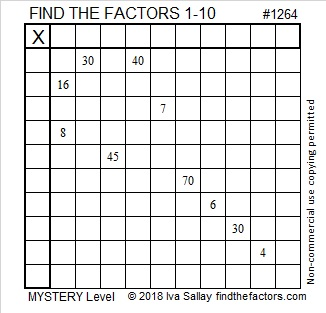# 1264 Dum Dums Mystery

Dum Dums have been around since 1924. If you go trick or treating, you will likely get at least one of these popular lollipops. Have fun solving the Dum Dums mystery puzzle I’ve made for you.Print the puzzles or type the solution in this excel file: 10-factors-1259-1270

Here are a few facts about the number 1264:

• 1264 is a composite number.
• Prime factorization: 1264 = 2 × 2 × 2 × 2 × 79, which can be written 1264 = 2⁴ × 79
• The exponents in the prime factorization are 4 and 1. Adding one to each and multiplying we get (4 + 1)(1 + 1) = 5 × 2 = 10. Therefore 1264 has exactly 10 factors.
• Factors of 1264: 1, 2, 4, 8, 16, 79, 158, 316, 632, 1264
• Factor pairs: 1264 = 1 × 1264, 2 × 632, 4 × 316, 8 × 158, or 16 × 79
• Taking the factor pair with the largest square number factor, we get √1264 = (√16)(√79) = 4√79 ≈ 35.552781264 is the sum of the first twenty-seven prime numbers. That’s all the prime numbers from 2 to 103.

This site uses Akismet to reduce spam. Learn how your comment data is processed.